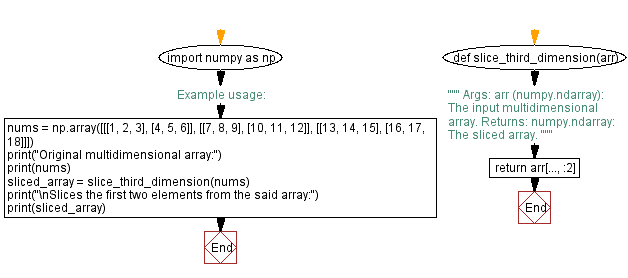﻿ Python Function: Slice first two elements from third dimension in multidimensional array

# Python Function: Slicing the third dimension of a multidimensional array

## Python ellipsis (...) Data Type: Exercise-3 with Solution

Write a Python function that takes a multidimensional array and slices the first two elements from the third dimension.

Sample Solution:

Code:

``````import numpy as np

def slice_third_dimension(arr):
"""
Args:
arr (numpy.ndarray): The input multidimensional array.

Returns:
numpy.ndarray: The sliced array.
"""
return arr[..., :2]

# Example usage:
nums = np.array([[[1, 2, 3], [4, 5, 6]],
[[7, 8, 9], [10, 11, 12]],
[[13, 14, 15], [16, 17, 18]]])
print("Original multidimensional array:")
print(nums)

sliced_array = slice_third_dimension(nums)
print("\nSlices the first two elements from the said array:")
print(sliced_array)
``````

Output:

```Original multidimensional array:
[[[ 1  2  3]
[ 4  5  6]]

[[ 7  8  9]
[10 11 12]]

[[13 14 15]
[16 17 18]]]

Slices the first two elements from the said array:
[[[ 1  2]
[ 4  5]]

[[ 7  8]
[10 11]]

[[13 14]
[16 17]]]
```

In the exercise above, the "slice_third_dimension()" function takes a NumPy multidimensional array as input and uses the ellipsis (...) to slice the first two elements from the third dimension. In the resulting sliced array, only the first two elements of the third dimension are preserved.

Flowchart:What is the difficulty level of this exercise?

Test your Programming skills with w3resource's quiz.

﻿

## Python: Tips of the Day

Summing a sequence of numbers (calculating the sum of zero to ten with skips):

```>>> l = range(0,10,2)
>>> sum(l)
20
```

We are closing our Disqus commenting system for some maintenanace issues. You may write to us at reach[at]yahoo[dot]com or visit us at Facebook